# P6 A car travels around a circular track at constant speed, as shown. It is observed...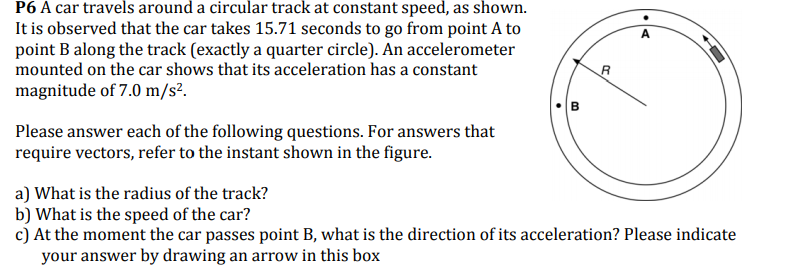P6 A car travels around a circular track at constant speed, as shown. It is observed that the car takes 15.71 seconds to go from point A to point B along the track (exactly a quarter circle). An accelerometer mounted on the car shows that its acceleration has a constant magnitude of 7.0 m/s2. Please answer each of the following questions. For answers that require vectors, refer to the instant shown in the figure. a) What is the radius of the track? b) What is the speed of the car? c) At the moment the car passes point B, what is the direction of its acceleration? Please indicate your answer by drawing an arrow in this box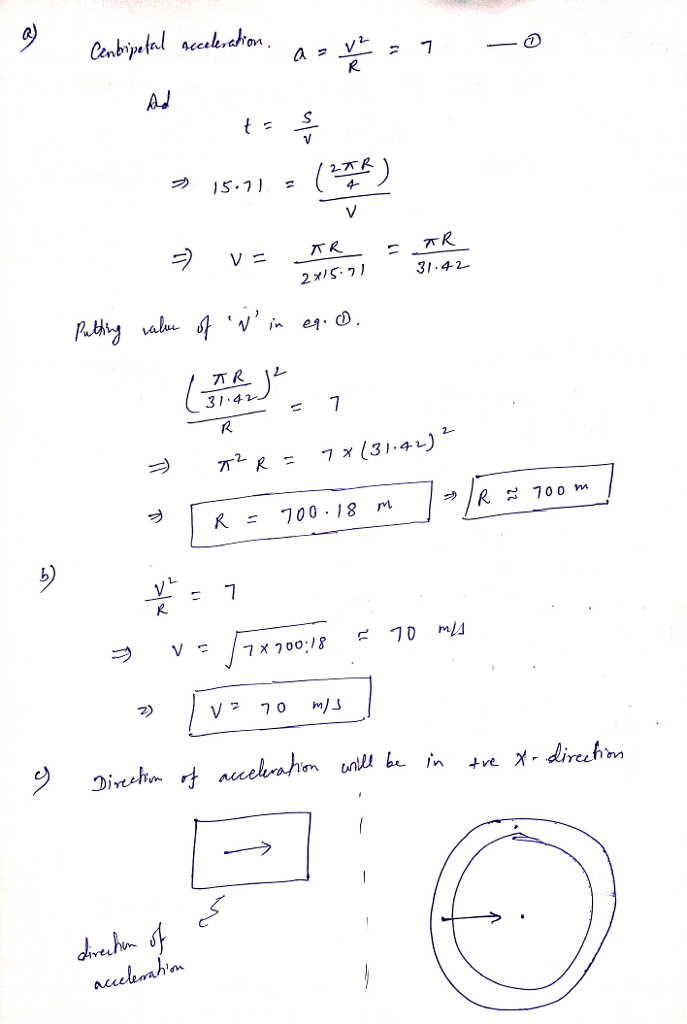please UPVOTE if you like the answer

#### Earn Coin

Coins can be redeemed for fabulous gifts.

Similar Homework Help Questions
• ### P6 A car travels around a circular track at constant speed, as shown. It is observed...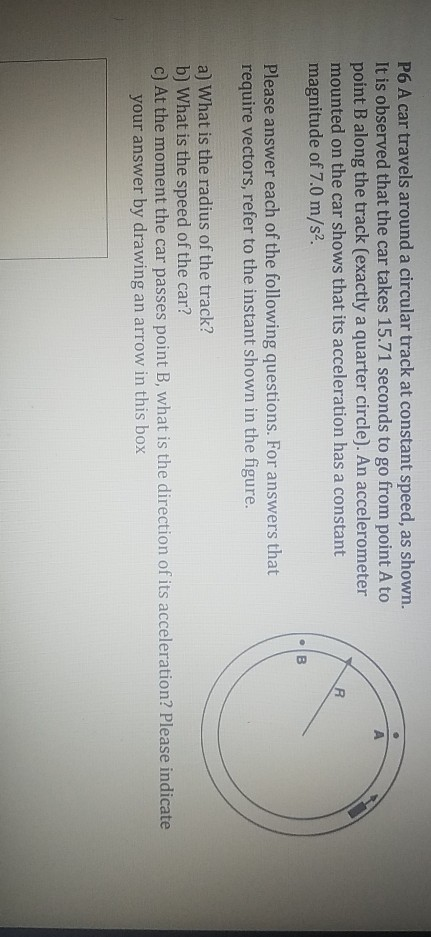P6 A car travels around a circular track at constant speed, as shown. It is observed that the car takes 15.71 seconds to go from point A to point B along the track (exactly a quarter circle). An accelerometer mounted on the car shows that its acceleration has a constant magnitude of 7.0 m/s2. Please answer each of the following questions. For answers that require vectors, refer to the instant shown in the figure. a) What is the radius of...

• ### A car travels at a constant speed around a circular track whose radius is 2.51 km....

A car travels at a constant speed around a circular track whose radius is 2.51 km. The car goes once around the track in 356 s. What is the magnitude of the centripetal acceleration of the car?

• ### A car travels at a constant speed around a circular track whose radius is 2.65 km. The car goes once around the track i...

A car travels at a constant speed around a circular track whose radius is 2.65 km. The car goes once around the track in 341 s. What is the magnitude of the centripetal acceleration of the car?

• ### A racing car travels with a constant tangential speed of 75.0 m/s around a circular track...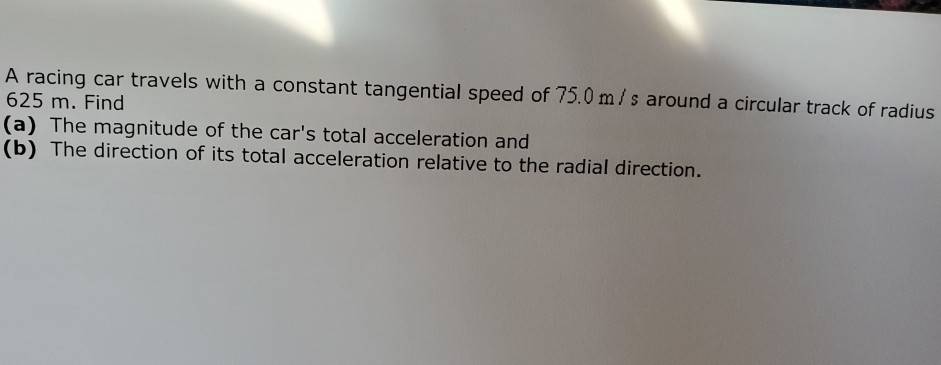A racing car travels with a constant tangential speed of 75.0 m/s around a circular track of radius 625 m. Find |(a) The magnitude of the car's total acceleration and |(b) The direction of its total acceleration relative to the radial direction.

• ### 1. An 800 kg car travels around the edge of a circular track of radius 400...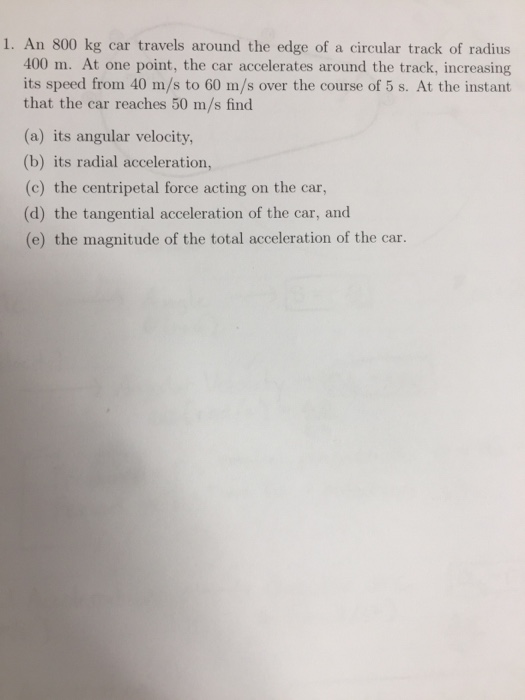1. An 800 kg car travels around the edge of a circular track of radius 400 m. At one point, the car accelerates around the track, increasing its speed from 40 m/s to 60 m/s over the course of 5 s. At the instant that the car reaches 50 m/s find (a) its angular velocity (b) its radial acceleration, (c) the centripetal force acting on the car, (d) the tangential acceleration of the car, and (e) the magnitude of the...

• ### A race car travels with a constant tangential speed of 81.3 m/s around a circular track...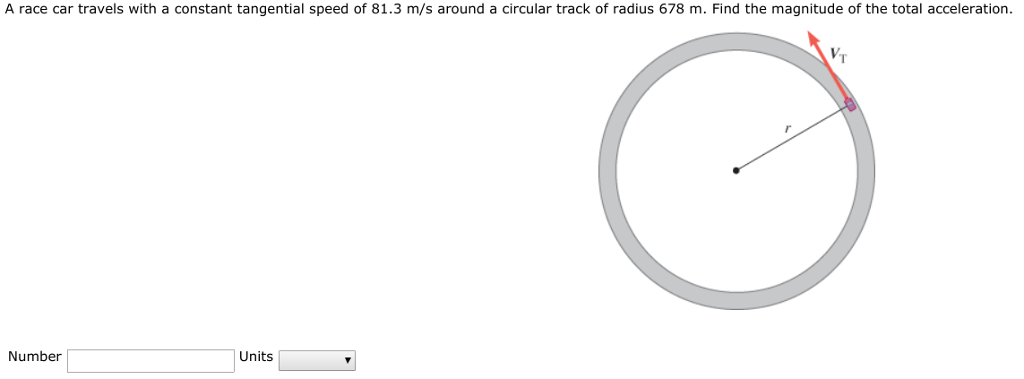A race car travels with a constant tangential speed of 81.3 m/s around a circular track of radius 678 m. Find the magnitude of the total acceleration VT Units Number

• ### The car travels at a constant speed on a circular banked track. The maximum possible speed of the...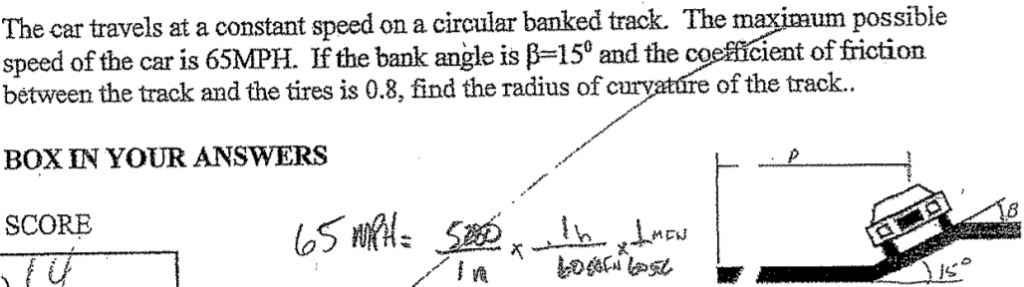The car travels at a constant speed on a circular banked track. The maximum possible speed of the car is 65MPH. If the bank angle is β=15° and the coefficient of friction bétween the track and the tires is 0.8, find the radius of curyatúre of the tra ck. BOX IN YOUR ANSWERS 8 The car travels at a constant speed on a circular banked track. The maximum possible speed of the car is 65MPH. If the bank angle is...

• ### A car is traveling around a horizontal circular track with radius r 190 m at a...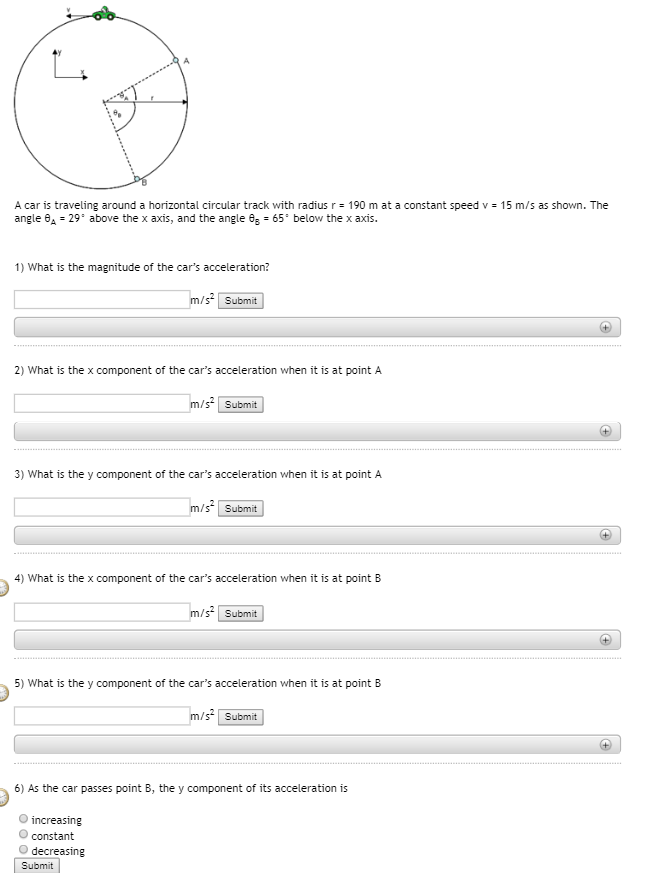A car is traveling around a horizontal circular track with radius r 190 m at a constant speed v angle θ' 29" above the x axis, and the angle es* 65° below the x axis. 15 m/s as shown. The 1) What is the magnitude of the car's acceleration? m/s Submit 2) What is the x component of the car's acceleration when it is at point A m/s Submit 3) What is the y component of the car's acceleration when...

• ### A race car goes around a level, circular track with a diameter of 1.00km at a constant speed of...

A race car goes around a level, circular track with a diameter of 1.00km at a constant speed of 84km/h. What is the car's centripetal acceleration in m/s squared?

• ### A racing car travels on a circular track with a radius of 200 m. If the...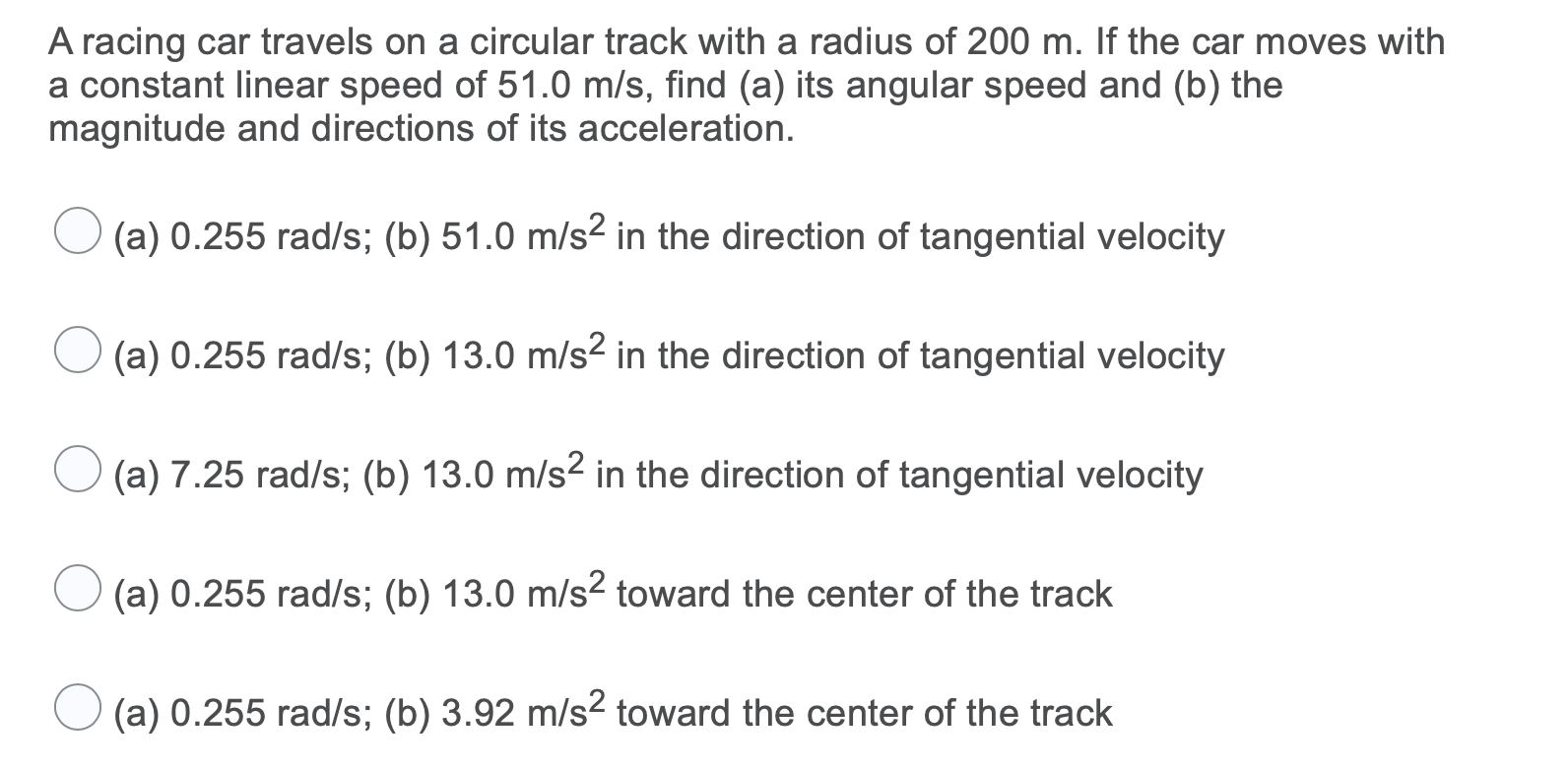A racing car travels on a circular track with a radius of 200 m. If the car moves with a constant linear speed of 51.0 m/s, find (a) its angular speed and (b) the magnitude and directions of its acceleration. (a) 0.255 rad/s; (b) 51.0 m/s2 in the direction of tangential velocity (a) 0.255 rad/s; (b) 13.0 m/s2 in the direction of tangential velocity (a) 7.25 rad/s; (b) 13.0 m/s2 in the direction of tangential velocity (a) 0.255 rad/s; (b)...

Free Homework App